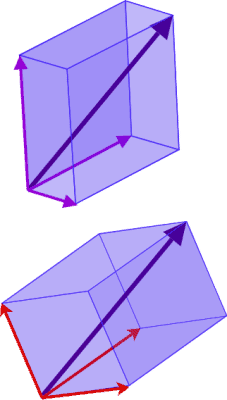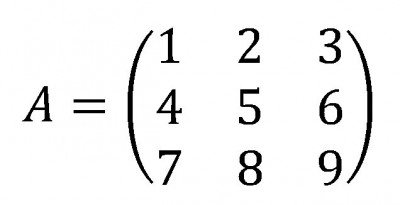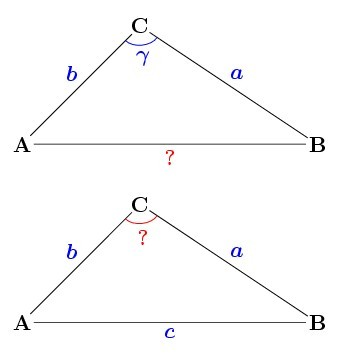# What Is A Dot Product? (12 Common Questions Answered)

Dot products are used in math and physics when working with vectors in 2, 3, or higher dimensions.  Dot products also come in handy when we are dealing with matrices.

So, what is a dot product?  A dot product takes 2 vectors as the input & returns a number as the output. For two vectors a = [a1, a2,…, an] and b = [b1, b2,…, bn], the dot product is a•b = a1b1 + a2b2 +…+ anbn. An alternative formula is a•b = ||a||*||b||*cos(C), where ||a|| is the magnitude of a & ||b|| is the magnitude of b.

Of course, the dot product will be zero if the vectors are orthogonal (that is, if there is a 90 degree angle between them).

In this article, we’ll talk about dot products and when they are well defined (or not).  We’ll also answer some common questions and show some examples of how to calculate dot products.

Let’s get started.

Hey! If you need to learn more about dot products and other math concepts for physics, check out this course:

Advanced Math For Physics: A Complete Self-Study Course

## What Is A Dot Product?

A dot product takes two vectors as inputs and combines them in a way that returns a single number (a scalar).

Given two vectors a and b in n-dimensional space:

• a = [a1, a2, … , an]
• b = [b1, b2, … , bn]

their dot product is given by the number:

• a•b = a1b1 + a2b2 + … + anbn

The dot product is also called the inner product of the vectors a and b.

It also has an alternative definition using the angle C between vectors a and b:

• a•b = ||a||*||b||*cos(C)

where ||a|| is the magnitude of vector a and ||b|| is the magnitude of vector b.  Remember that the magnitude of a vector x = [x1. x2, … , x] is given by the square root of the dot product of x with itself, or:

• ||x|| = √(x•x)
• ||x|| = √(x12 + x22 + … + x2)

Another more familiar name for the magnitude of a vector in 1D, 2D, or 3D is its length.

### How Dot Product Works

Let’s take a look at some examples to see how dot product works.

#### Example 1: Dot Product For Two Vectors In Two Dimensions

Let’s say we want to take the dot product of the vectors

• a = [2, 4]
• b = [3, -1]

The dot product formula would give us:

• a•b = a1b1 + a2b2 + … + anbn
• a•b = a1b1 + a2b2 [n = 2, since there are only 2 dimensions]
• a•b = (2)(3) + (4)(-1)
• a•b = 6 + -4
• a•b = 2

So, the dot product of the vectors a and b is 2.

Note that we can now use the other dot product formula to find the angle C between the vectors a and b:

• a•b = ||a||*||b||*cos(C)
• 2 = ||[2, 4||*||3, -1||*cos(C)
• 2 = (√(22 + 42) + √(32 + (-1)2))*cos(C)
• 2 = (√20 + √10)cos(C)
• 2 = 7.63cos(C)
• 2 / 7.63 = cos(C)
• 0.262 = cos(C)
• arccos(0.262) = C

So, the angle between the two vectors is 1.306 radians, or 74.8 degrees.

#### Example 2: Dot Product For Two Vectors In Three Dimensions

Let’s say we want to take the dot product of the vectors

• a = [1, -2, 8]
• b = [4, 5, -3]

The dot product formula would give us:

• a•b = a1b1 + a2b2 + … + anbn
• a•b = a1b1 + a2b2 + a3b3 [n = 3, since there are only 3 dimensions]
• a•b = (1)(4) + (-2)(5) + (8)(-3)
• a•b = 4 – 10 – 24
• a•b = -30

So, the dot product of the vectors a and b is -30.We can also use dot product to find the angle between two vectors in 3 dimensions.

### Can You Dot Product A Scalar & A Vector?

You cannot dot product a scalar and a vector.  The definition of a dot product requires the input of two vectors a and b, both of which have the same dimension of n.

The dot product of a scalar and a vector is undefined.

However, you can multiply a vector a by a scalar k as follows:

• ka
• =k[a1, a2, … , an]
• =[ka1, ka2, … , kan]

Note that this is not the same as the dot product.

### Is Dot Product Commutative?

Dot product is commutative, since multiplication in the real numbers is commutative.  We can prove it as follows:

• a•b
• = a1b1 + a2b2 + … + anbn  [by definition of dot product of a and b]
• = b1a1 + a2b2 + … + anbn  [since a1b1 = b1a1, by commutativity of multiplication of real numbers]
• = b1a1 + b2a2 + … + anbn  [since a2b2 = b2a2, by commutativity of multiplication of real numbers]
• = b1a1 + b2a2 + … + bnan  [since anbn = bnan, by commutativity of multiplication of real numbers]
• =b•a  [by definition of dot product of b and a]

So, a•b = b•a for two vectors a and b with the same dimensions, meaning dot product is commutative.

### Is Dot Product Associative?

Dot product is not associative, since the products are not well-defined in this case (as we mentioned earlier, we cannot take the dot product of a scalar and a vector).  For example, let’s take three n-dimensional vectors a, b, and c.

First, we can find a•b, which is simply a scalar (a number).  However, we cannot take the dot product of this scalar with the vector c, which means (a•b)•c is not well defined.

Likewise, we can find b•c, which is simply a scalar (a number).  However, we cannot take the dot product of the vector a with this scalar, which means a•(b•c) is not well defined.

Since both (a•b)•c and a•(b•c) are not well defined, we know that they cannot be equal.  So, dot product is not associative.

### Can You Dot Product Three Vectors?

You cannot dot product three vectors in n dimensions.  The reason is similar to the above reasoning for associativity: as we mentioned earlier, we cannot take the dot product of a scalar and a vector.

If you have three n-dimensional vectors a, b, and c, the dot product a•b is defined, and we can calculate it.  However, the dot product of a•b with c is not defined, since a•b is a scalar and c is a vector in n dimensions.

### Can You Dot Product Vectors Of Different Dimensions?

You cannot take the dot product of vectors with different dimensions.  The definition of the dot product requires both vectors a and b to have the same dimension of n (n can be any number, but it must be the same for both vectors).

### Can You Distribute Dot Product?

We can distribute the dot product as long as we have sums and products of vectors with the same dimensions.

For example, if a, b, and c are vectors in n dimensions, then the following formula is true:

• a•(b + c) = a•b + a•c

Note that when we add two vectors in n dimensions (b and c), we get another vector in n dimensions (b + c).  Then, we can take the dot product of a and b + c, since both vectors have n dimensions.

The proof is shown below:

• a•(b + c)
• = a•([b1, b2, … , bn] + [c1, c2, … , cn])
• = a•([b1 + c1, b2 + c2, … , bn + cn])
• = a1[b1 + c1] + a2[b2 + c2] + … + an[bn + cn]
• = (a1b1 + a1c1) + (a2b2 + a2c2) + … + (anbn + ancn)
• = (a1b1 + a2b2 + … + anbn) + (a1c1 + a2c2 + … + ancn)
• = a•b + a•c

### Can You Dot Product A Matrix & A Vector?

You can think of multiplying a matrix by a vector as a series of dot products.  In effect, you are taking the dot product of the vector with each row of the matrix, and recording the result as an entry in the product vector.When you multiply a matrix by a vector, you are using a series of dot products to find the resulting matrix (which is another vector).

However, the dimensions must match for the multiplication to be well defined.  If the matrix has dimensions m by n, then the vector must have dimension n (an n by 1 matrix).

For example, if M is a 4×3 matrix (4 rows, 3 columns) and A is a 3×1 matrix (3 rows, 1 column – a vector in 3 dimensions), then the product would be a 4×1 matrix (4 rows, 1 column – a vector in 4 dimensions).

If the rows of the matrix M are M1, M2, M3, and M4, then the entries of the resulting 4×1 matrix would be dot products:

• M1•A
• M2•A
• M3•A
• M4•A

### Can Dot Product Be Negative?

Dot product can be negative, since we are not taking absolute value when we add products of coordinates from the vector inputs.

For example, recall the example from earlier: the dot product of a•b = [1, -2, 8]•[4, 5, -3] is -30.

### Can Dot Product Be Greater Than 1?

Dot product can be greater than 1, since the vectors can have any length.

For example, recall the example from earlier: the dot product of a•b = [2, 4]•[3, -1] is 2, which is greater than 1.

If the vectors a and b both have lengths of at most 1, then their dot product cannot be greater than 1.  This is because the cosine of any angle is between -1 and 1, meaning |cos(C)| <= 1 for any angle C.

The dot product formula from before is:

• a•b = ||a||*||b||*cos(C)

Taking the absolute value of both sides and using inequalities gives us:

•  |a•b| <= 1*1*1
• |a•b| <= 1

So in this case, the dot product cannot be greater than 1.

### Can Dot Product Be Zero?

Dot product can be zero in some cases.  For example, if one or both vectors has zero for every coordinate, then the dot product is zero.

In fact, if at least one of the vectors has a zero coordinate in each dimension, then the dot product is zero.

The dot product is also zero if the angle between the two vectors a and b is 90 degrees (that is, they are orthogonal vectors).  This is because the dot product formula containing cosine would give us cos(90), which is zero.If two vectors a and b are orthogonal, then the angle between them is 90 degrees, and their dot product is zero.

#### Example 1: A Dot Product Of Two Vectors That Is Zero

Consider the vectors

• a = [2, 0]
• b = [0, 7]

The dot product formula would give us:

• a•b = a1b1 + a2b2
• a•b = (2)(0) + (0)(7)
• a•b = 0 + 0
• a•b = 0

So, the dot product of the vectors a and b is 0.

#### Example 2: A Dot Product Of Two Vectors That Is Zero

Consider the vectors

• a = [3, 13, -4]
• b = [7, -1, 2]

The dot product formula would give us:

• a•b = a1b1 + a2b2 + a3b3
• a•b = (3)(7) + (13)(-1) + (4)(2)
• a•b = 21 – 13 + 8
• a•b = 0

So, the dot product of the vectors a and b is 0.

### What Are Orthogonal Vectors?

Orthogonal vectors have a 90 degree (π/2 radians) between them.  This means that if a and b are orthogonal, then their dot product is zero, since:

• a•b = ||a||*||b||*cos(C)
• a•b = ||a||*||b||*cos(90)  [since the angle between a and b is 90 degrees]
• a•b = ||a||*||b||*0  [since the cosine of 90 degrees is zero]
• a•b = 0

## Conclusion

Now you know what a dot product is and what it means.  You also know the answers to some common questions about dot product and when it is (or is not) well defined.